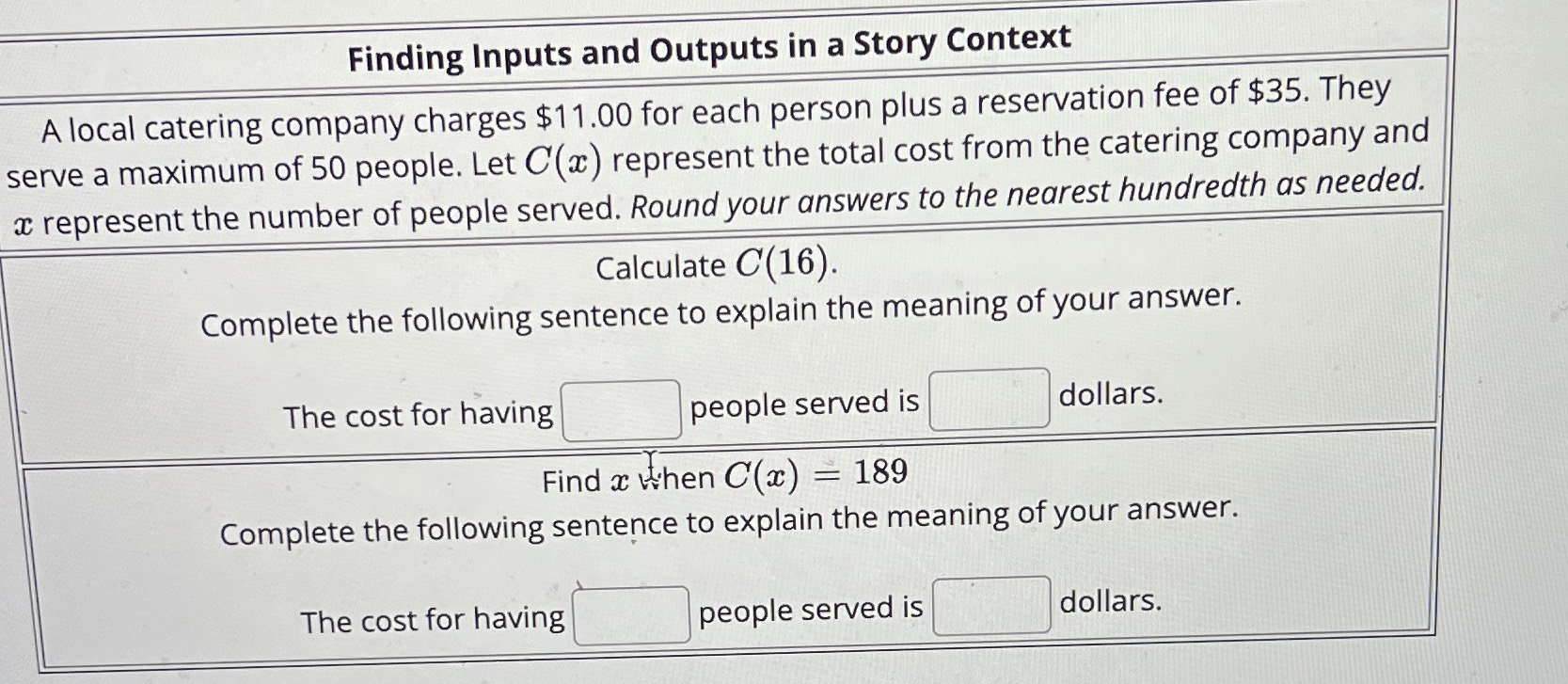### ¿Todavía tienes preguntas de matemáticas?

Pregunte a nuestros tutores expertos
Algebra
PreguntaA local catering company charges $$\ 11.00$$ for each person plus a reservation fee of $$\ 35 .$$ They serve a maximum of $$50$$ people. Let $$C ( x )$$ represent the total cost from the catering company and $$x$$ represent the number of people served. Round your answers to the nearest hundredth as needed.

Calculate $$C ( 16 )$$:

The cost for having $$\square$$ people served is $$\square$$ dollars.

Find $$x$$ when $$C ( x ) = 189$$

The cost for having $$\square$$ people served is $$\square$$ dollars.
$$16,\ 211\\14,\ 189$$